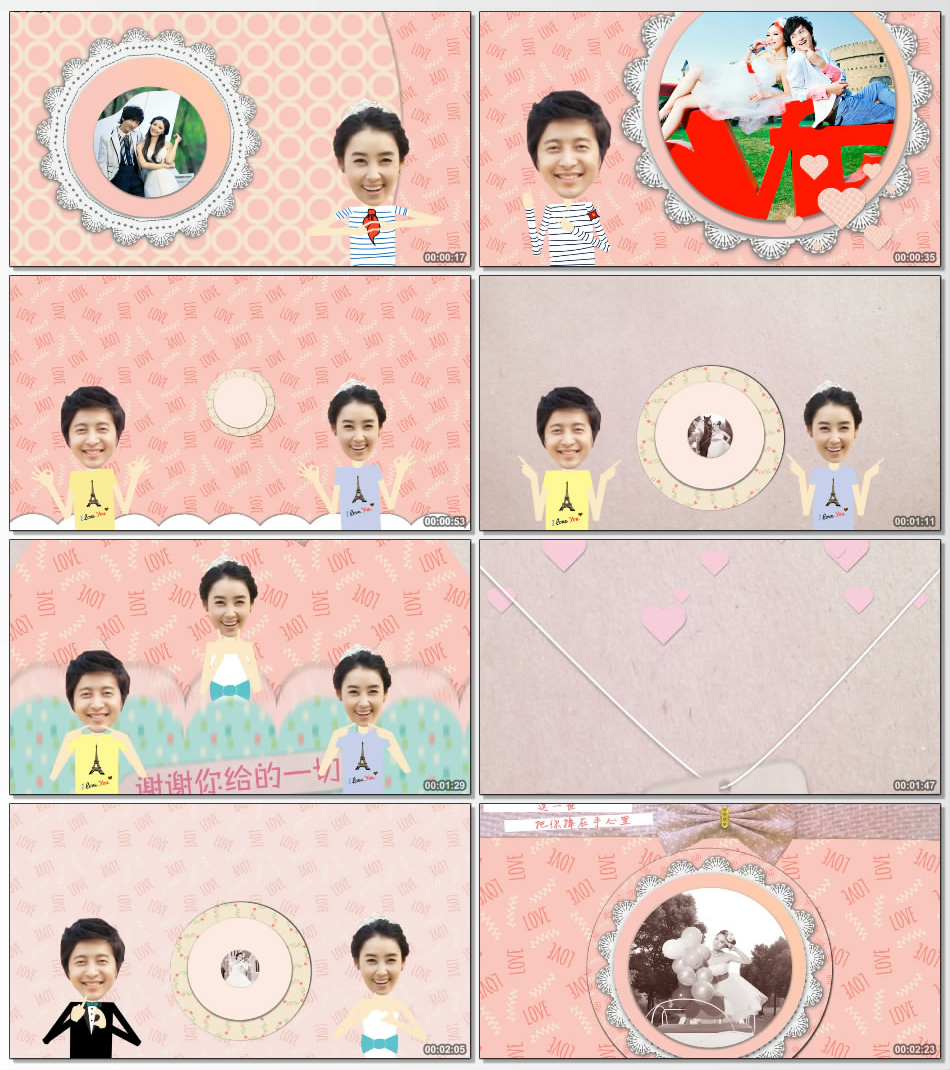# 可爱颂Guiyomit1=秦朝&苏姬
t2=我们的爱情
t3=终于等到这一天
t4=这一天将成为我们最美记忆
t5=从相遇那天起
t6=幸福的开始
t7=从今天起 我们一起努力幸福
t8=你和阳光都在
t9=每天早晨起来
t10=这就是我想要的生活
t11=谢谢你给的一切
t12=和你在一起 我的世界充满色彩
t13=我们彼此的
t14=执着与坚定
t15=才有今天的十指相扣
t16=谢谢你给的一切

t17=这就是我想要的生活

t18=对……

t19=从今天起

t20=2015.12.18
t21=当我遇到你
t22=当爱情遇到爱情
t23=幸福就是理所当然
t24=亲爱的
t25=我们一起珍惜
t26=从今天起 我们一起努力幸福
t27=肩并肩永远在一起
t28=幸福的每一天
t29=我们一起慢慢变老
t30=直到老的哪都去不了
t31=这一天 我为你戴上幸福的戒指
t32=这一世
t33=把你捧在手心里
t34=亲爱的
t35=我们一起珍惜
t36=盛大婚礼
t37=即将开始
t38=苏姬
t39=秦朝